# SQL Query to Convert UTC to Local Time Zone

• Last Updated : 19 May, 2021

In this article, we will cover how to convert UTC to the local time zone for the different times of UTC in the database. Now, let’s execute a query that converts the UTC to local time zone using MSSQL as our database in detail step-by-step.

Step 1: Creating a database time_converter by using the following SQL query as follows.

`CREATE DATABASE time_converter;`

Step 2: Using the database time_converter using the following SQL query as follows.

`USE time_converter;`

Step3: Creating table times with 2 columns using the following SQL query as follows.

Let us create a table with index and DATETIME as a datatype.

```CREATE TABLE times
(Sno INT,
date_time DATETIME);```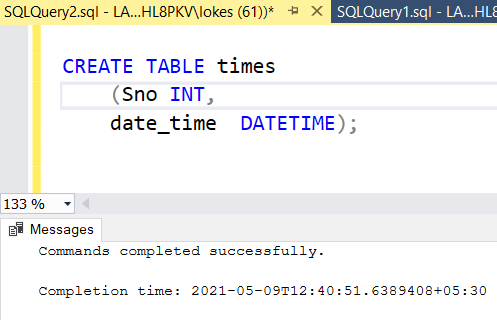Step 4: To view the description of the tables in the database using the following SQL query as follows.

`EXEC sp_columns times;`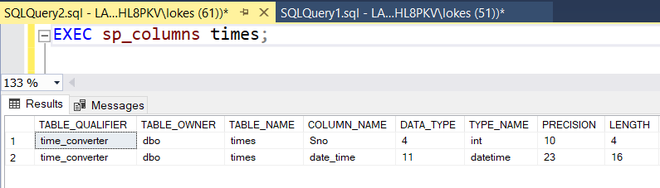Step 5: Inserting rows into times table using the following SQL query as follows.

```INSERT INTO times VALUES
(1,'2021-03-01 12:00:00'),
(2,'2021-04-01 09:30:00'),
(3,'2021-02-05 10:50:00'),
(4,'2021-04-18 08:50:00');```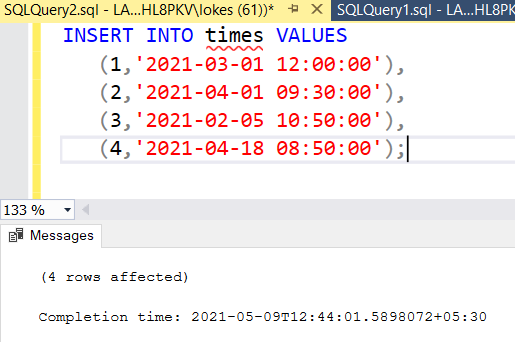Step 6: Viewing the table times after inserting rows by using the following SQL query as follows.

`SELECT * FROM times;`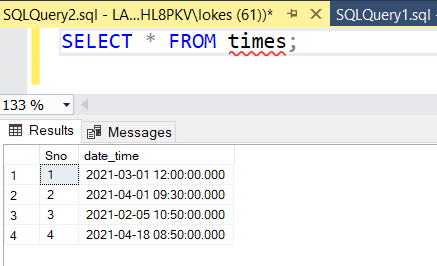Query 1: Query to convert current time from UTC to local IST (Indian Standard Time)

Note: India’s time zone is 5hours and 30 minutes ahead of UTC.

Syntax:

```SELECT colum_name,
CONVERT(datetime,
SWITCHOFFSET(CONVERT(DATETIMEOFFSET, column_name),
DATENAME(TZOFFSET, SYSDATETIMEOFFSET())))
AS general_name```
```SELECT
CONVERT(datetime,
SWITCHOFFSET(CONVERT(DATETIMEOFFSET, GETUTCDATE()),
DATENAME(TZOFFSET, SYSDATETIMEOFFSET())))
AS LOCAL_IST;```

Here, the GETUTCDATE() function can be used to get the current date and time UTC. Using this query the UTC gets converted to local IST.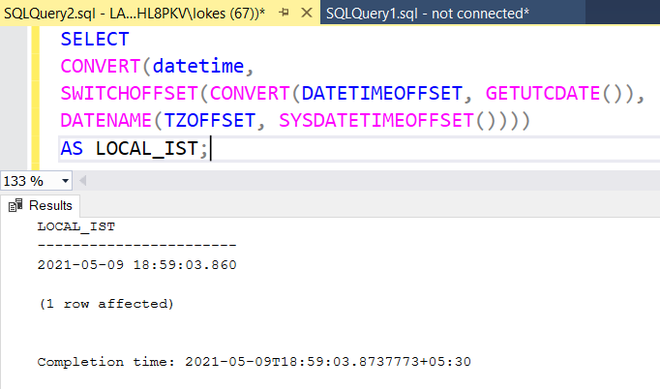Query 2: Query to convert all the times in times table to local IST from UTC.

Method 1:

In this method, the UTC times in the table get converted to local Indian standard time. To get the UTC converted to IST, UTC must be added with “+05:30” the same gets added in the output.

Syntax:

```SELECT colum_name,
CONVERT(datetime,
SWITCHOFFSET(CONVERT(DATETIMEOFFSET, column_name),
DATENAME(TZOFFSET, SYSDATETIMEOFFSET())))
AS general_name
FROM table_name;```
```SELECT date_time,
CONVERT(datetime,
SWITCHOFFSET(CONVERT(DATETIMEOFFSET, date_time),
DATENAME(TZOFFSET, SYSDATETIMEOFFSET())))
AS LOCAL_IST
FROM times;```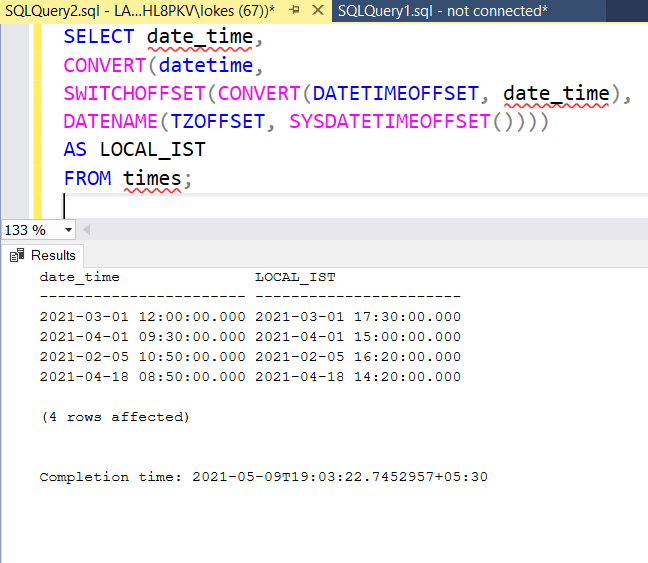Method 2:

In this method, the difference between the present date-time(GETDATE()) and UTC (GETUTCDATE()) date time gets added to the dates in date_time column.

Syntax:

```SELECT
column_name,
DATEADD(MI, DATEDIFF(MI, GETUTCDATE(), GETDATE()), date_time)
AS LOCAL_IST
FROM times;```
```SELECT date_time,
DATEADD(MI, DATEDIFF(MI, GETUTCDATE(), GETDATE()), date_time)
AS LOCAL_IST
FROM times;```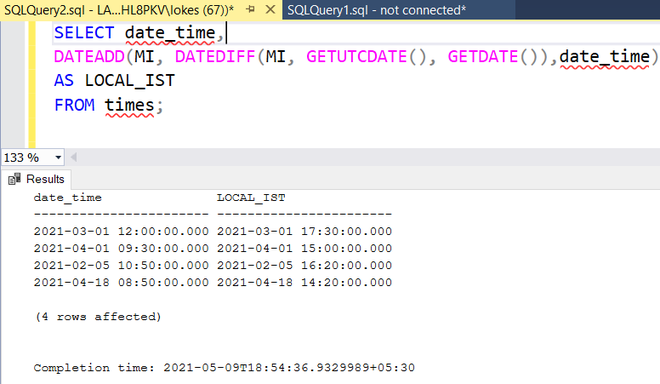My Personal Notes arrow_drop_up